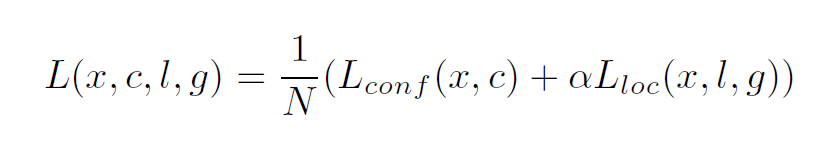top of page
Search
• Xuehao Liu

# SSD Object Detection Reading Notes

Updated: Mar 14, 2019

With the powerful CNNs that can capture image features, we can finish more interesting tasks such as object detection. The following paragraphs are my reading notes of the paper: 'SSD: Single Shot MultiBox Detector'.

The idea of SSD

In the first place, the CNNs are used in the image classification task. If we push an image into a CNN, the CNN will give us a probability about the class of that image. The convenience of CNNs is that we do not have to design the image feature, because the CNNs will be able to detect those image features after the training process. The image feature of traditional object detection method is quite complicated. So why do not use the CNNs to detect the object?

The first object detection CNN, RCNN, is a very intuitive idea. If we can do the classification task on the whole image, we can select the region of one image containing the object, and classify that region. And the answer is positive. We can do this, but we still need to propose thousands of regions so that we can ensure that we do not miss targets. And it takes a relatively long running time.

The second thought of this idea is that if we can detect different original regions of an image, we should be able to use the regions on a feature map that extracted by a CNN, such as VGG net. I think maybe this is where Single Shot Detector(SSD) comes from.

StructureThe overall structure of SSD
• Backbone

The base feature map of SSD is the VGG-16 net. VGG-16 is trained to do the classification job. The top 5 error of this network on a 1000-class ImageNet dataset is 7.5%. We can say that it can capture most of the image features. Using the VGG-16 as the backbone, the network of SSD starts with the Conv5_3 layer in VGG-16 net. Layer Conv5_3 is just the last weighted layer, and this can be seen as the input of SSD network.

• Layers

SSD contains 10 weighted convolutional layers. The parameters have been shown above. The output size of the last layer is 1*1*256. The network will not stop at this point. If we need to detect the object in different sizes, we need to capture different level/layer/size of features. The way of doing this is to do a 3*3 convolution on the feature map. This operation will become the actual output of the whole network. The channel number of this output is 4 or 6 * (class +4). In this place, 4 or 6 is the number of bounding boxes. (class +4) is the probability of every class on that bounding box, plus the 4 coordinates can locate the exact place of that bounding box. The detail of this part will be discussed in the following paragraphs.

Which exact layer is used to produce the output is shown in the picture. The Detection layer on the picture is the concatenation of those outputs. The size of this layer is 8732 per class because 8732 = (38*38 + 3*3 +1) * 4 + (19*19 + 10*10 + 5*5) * 6. The size of this layer depends on which layer you want to choose as the out put of the network.

So the output of the Detection layer is 8732*(class +4). But we are not going to compute the loss using so many boxes. These results will be passed into a non-maximum suppression (NMS) layer. This step is called Inference. In this inference, since most of those 8732 boxes will not be the target boxes, a threshold of 0.01 can filter those negative boxes. After that, we eliminate other overlapping boxes by applying NMS on these positive boxes. There will be 200 boxes remaining, and the loss function is computed on these 200 boxes.

Loss functionOverall loss functionLocation lossConfident loss

So the loss function is quite straight forward. It is consisted with weighted confident loss and location loss.

g is groud truth box. d is detected box. c is the coorasponding class. cx and cy is the coorinate of the center of that box. w and h is the with and height of the box.

The comfodent loss is the softmax multi-class loss.

Detection boxes

The location of all 8732 boxes are decided by the anchors on the feature maps. If we select a feature map which has the size m*n*channel, we will have m*n anchors.